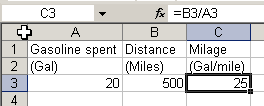## Subscribe to our mailing list

* indicates required

Audit !!!

Check out our RefTreeAnalyser
the ultimate Excel formula auditing tool.

Trainings

Excel VBA Masterclass (English)
Excel VBA for Financials (Dutch)

Third party tools

FastExcel
The best tool to optimise your Excel model!

What has changed?

This Excel compare tool is fast, reliable, and easy to use. Compare two Excel files now and see for yourself!
Home > English site > Articles > Formula Wrapper > Formula Wrapper# Wrapping Formulas In An Error Test

## First version

Oftentimes I have a range filled with formulas that as such work correctly, but need an error checking mechanism. Take for example the following simple situation:If cell A3 contains no data or a zero, the result in C3 will be #DIV/0!

If you don't want the errors to show, the solution is simple: test for zero:

=IF(A3=0,"",B3/A3)

But I tend to have much more complicated formulas, in which it is not always just one cell that might cause an error of some sort, so I need to wrap the entire formula in an error check. I thought it might be useful to have a generic little tool that lets you specify how to wrap the current function in a cell with an error test.

Here is the first try at a subroutine:

Sub ChangeFormulas()
Dim oCell As Range
Dim sFormula As String
Dim sInput As String
Static sFormulaTemplate As String
If sFormulaTemplate = "" Then
sFormulaTemplate = "=IF(ISERROR(_form_),"""",_form_)"
End If
sInput = InputBox("Enter base formula", , sFormulaTemplate)
If sInput = "" Then Exit Sub
sFormulaTemplate = sInput
For Each oCell In Selection
sFormula = Replace(sFormulaTemplate, "_form_", Right(oCell.Formula, Len(oCell.Formula) - 1))
oCell.Formula = sFormula
Next
End Sub

So how does this work?

You select the cells with the formulas that need worked over.
Then run the sub. An Input box is shown with a default template to change the formula:

=IF(ISERROR(_form_),"",_form_)

What this means is that the current formula in the cell is going to be placed at each location where it says "_form_". Edit the formula template to match your need and click OK.

Because sFormulaTemplate is declared as Static, the utility remembers the template for a next time you use it.